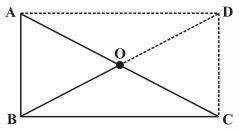# ABC is a right-angled triangle and O is the mid-point of the side opposite to the right angle. Explain why O is equidistant from A, B and C.Solution

Construction: Draw two lines AD and DC such that AD II BC and AB II DC

AD = BC , AB = DC

Since, ABCD is a rectangle.

Opposite sides are parallel and equal to each other and all the interior angles are right angles.

The property of rectangle states that the diagonals are of equal length and bisect each other.

Hence, AO = OC = BO = OD.

Thus, O is equidistant from A, B and C.